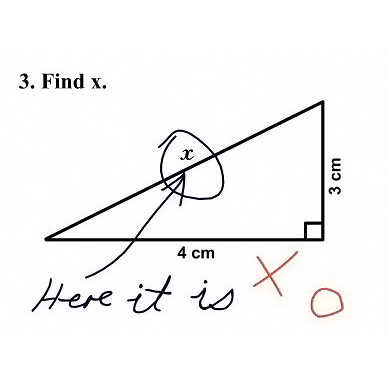# Wonky Social Media Mathit’s popular to circulate math problems on social media. The solutions proposed in the comments are often spectacularly wrong.

What is the answer to 1+1+1+1+1+1+1+1+1+1-1+1+1+1+1+1+1×0 = ?

The answer is 14! I keep seeing this circulate the internet, generating pages of comments, people brazenly stating an incorrect answer as if it were the gospel truth and backing it with the most uneducated reasoning. Sometimes they even claim to be math majors (and they won’t be for long should this in fact be true).

I would like to put this to rest. The answer is 14, get over it. There is an established order for calculating equations, the order of operations, and that’s simply how it is.

It seems that the most common wrong answer is 0, because “anything times 0 is 0.” Well yes, but only the last 1 is being “times’d” by 0. I would like to use storytelling to explain.

Lets say that you have a job which pays you \$20/hour and that you work 8 hour days. Now it so happens that you take Friday off this week, so you work 0 hours on Friday. Below is a reasonable hour/wages log for this week:

So now, if you wanted to find out how much you have earned this week, you would add up the amounts earned each day:

```Amount Earned = \$20 x 8 + \$20 x 8 + \$20 x 8 + \$20 x 8 + \$20 x 0
= \$160 + \$160 + \$160 + \$160 + \$20 x 0
= ???```

Rest assured, you would earn \$640 that week, and not a juicy zero for taking the Friday off.